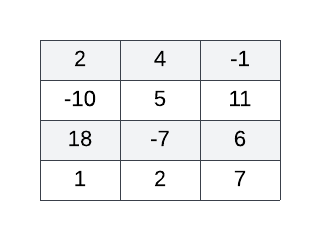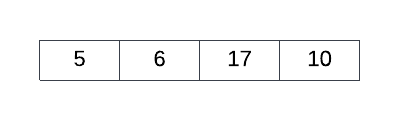Login or Create an Account to view the mark scheme, comment, and add to a test
Given a 2D array called SCORES[row index][column index] of size 4x3 (rows x columns) where each row contains the game scores for a different computer game, construct an algorithm to output a new 1D array called TOTALS containing the total score of each computer game, that is, the sum of each row in SCORES (in row order).

For example, consider the following 2D array:The correctly functioning algorithm applied to the 2D array above yields the following 1D array:HomeThe Right Test - Fast